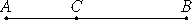# Proposition 73

If from a rational straight line there is subtracted a rational straight line commensurable with the whole in square only, then the remainder is irrational; let it be called an apotome.

From the rational straight line AB let the rational straight line BC, commensurable with the whole in square only, be subtracted.

I say that the remainder AC is the irrational straight line called apotome.X.11

Since AB is incommensurable in length with BC, and AB is to BC as the square on AB is to the rectangle AB by BC, therefore the square on AB is incommensurable with the rectangle AB by BC.

But the sum of the squares on AB and BC is commensurable with the square on AB, and twice the rectangle AB by BC is commensurable with the rectangle AB by BC.

And, inasmuch as the sum of the squares on AB and BC equal twice the rectangle AB by BC together with the square on CA, therefore the sum of the squares on AB and BC is also incommensurable with the remainder, the square on AC.

X.Def.I.4

But the sum of the squares on AB and BC is rational, therefore AC is irrational. Let it be called an apotome.

Q.E.D.

## Guide

This proposition is used very frequently in the rest of Book X starting with X.75. It is also used in propositions XIII.6 and XIII.11.